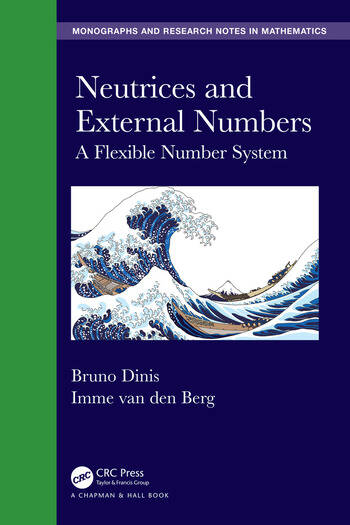# Neutrices and External Numbers: A Flexible Number System

## 1st Edition

Bruno Dinis, Imme van den Berg

Chapman and Hall/CRC
Published June 24, 2019
Reference - 342 Pages - 4 B/W Illustrations
ISBN 9781498772679 - CAT# K29518
Series: Chapman & Hall/CRC Monographs and Research Notes in Mathematics

was \$174.95

USD\$139.96

SAVE ~\$34.99

FREE Standard Shipping!

## Preview

### Summary

Neutrices and External Numbers: A Flexible Number System introduces a new model of orders of magnitude and of error analysis, with particular emphasis on behaviour under algebraic operations. The model is formulated in terms of scalar neutrices and external numbers, in the form of an extension of the nonstandard set of real numbers. Many illustrative examples are given. The book starts with detailed presentation of the algebraic structure of external numbers, then deals with the generalized Dedekind completeness property, applications in analysis, domains of validity of approximations of solutions of differential equations, particularly singular perturbations. Finally, it describes the family of algebraic laws characterizing the practice of calculations with external numbers.

Features

• Presents scalar neutrices and external numbers, a mathematical model of order of magnitude within the real number system.
• Outlines complete algebraic rules for the neutrices and external numbers
• Conducts operational analysis of convergence and integration of functions known up to orders of magnitude
• Formalises a calculus of error propagation, covariant with algebraic operations
• Presents mathematical models of phenomena incorporating their necessary imprecisions, in particular related to the Sorites paradox